ALL

Page: (Previous)   1  2  3  4  5  6  (Next)
ALL

### M

#### MAT-HS.G-CO.11.a

 MAT-HS Targeted Standards(G) Concept: Geometry(CO) Domain: CongruenceCluster: Prove geometric theorems MAT-HS.G-CO.11.b Prove theorems about parallelograms. (Focus on Prove for G-CO.11) (Theorems include but are not limited to: opposite sides are congruent, opposite angles are congruent, the diagonals of a parallelogram bisect each other, and conversely, rectangles are parallelograms with congruent diagonals.)

• I can
• I can

• I can
• I can

• I can
• I can

• I can
• I can

## Proficiency Scale

 Score Description Sample Activity Advanced In addition to Score 3.0, the student demonstrates in-depth inferences and applications regarding more complex material that go beyond end of instruction expectations. Analyze a proof with errors and make corrections - 3.5 In addition to Score 3.0 performance, the student demonstrates in-depth inferences and applications regarding the more complex content with partial success. Proficient “The Standard.” The student demonstrates no major errors or omissions regarding any of the information and processes that were end of instruction expectations. The student can perform all the actions for each concept listed below: Identify relevant information Arrange reasoning in a logical sequence Support reasoning using appropriate justifications (theorems, postulates, definitions, etc.)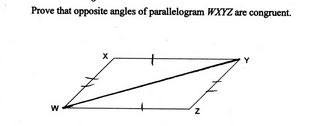2.5 The student demonstrates no major errors or omissions regarding the simpler details and processes (Score 2.0 content) and partial knowledge of the more complex ideas and processes (Score 3.0 content). Progressing The student demonstrates no major errors or omissions regarding the simpler details and processes but exhibits major errors or omissions regarding the more complex ideas and processes (Score 3.0 content). Partially prove but reasoning has gaps, steps missing, incorrect reasoning, etc. Recall theorems, definitions, and postulates Fill out justification given a statement - 1.5 The student demonstrates partial knowledge of the simpler details and processes (Score 2.0 content) but exhibits major errors or omissions regarding the more complex ideas and procedures (Score 3.0 content). Beginning With help, the student demonstrates a partial understanding of some of the simpler details and processes (Score 2.0 content) and some of the more complex ideas and processes (Score 3.0 content). - 0.5 With help, the student demonstrates a partial understanding of some of the simpler details and processes (Score 2.0 content) but not the more complex ideas and processes (Score 3.0 content). 0.0 Even with help, the student demonstrates no understanding or skill. -

## Resources

 Web Vocab

#### MAT-HS.G-CO.11.b

 MAT-HS Targeted Standards(G) Concept: Geometry(CO) Domain: CongruenceCluster: Prove geometric theorems MAT-HS.G-CO.11.b Apply theorems about parallelograms. Theorems include but are not limited to: opposite sides are congruent, opposite angles are congruent, the diagonals of a parallelogram bisect each other, and conversely, rectangles are parallelograms with congruent diagonals. (Focus is on Application for G-CO.11B)

• I can
• I can

• I can
• I can

• I can
• I can

• I can
• I can

## Proficiency Scale

 Score Description Sample Activity Advanced In addition to Score 3.0, the student demonstrates in-depth inferences and applications regarding more complex material that go beyond end of instruction expectations. - 3.5 In addition to Score 3.0 performance, the student demonstrates in-depth inferences and applications regarding the more complex content with partial success. Proficient “The Standard.” The student demonstrates no major errors or omissions regarding any of the information and processes that were end of instruction expectations. Apply theorems (postulates, definitions, etc.) about parallelograms to solve problems Justify how to set up a problem using theorems (postulates, definitions, etc.) about parallelograms Solve for x and y.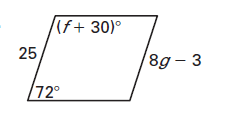2.5 The student demonstrates no major errors or omissions regarding the simpler details and processes (Score 2.0 content) and partial knowledge of the more complex ideas and processes (Score 3.0 content). Progressing The student demonstrates no major errors or omissions regarding the simpler details and processes but exhibits major errors or omissions regarding the more complex ideas and processes (Score 3.0 content). Identify the theorem, postulate, or definition used to set up and solve a problem Able to recognize and recall important vocabulary such as:                 opposite sides                 opposite angles                 diagonals                 congruent triangles                  perpendicular                 CPCTC Students are able to find the missing measurements  in a parallelogram given some basic information Can determine if a given figure is a parallelogram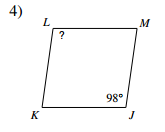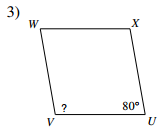Identify if the given figure is a parallelogram.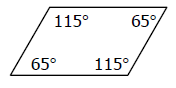1.5 The student demonstrates partial knowledge of the simpler details and processes (Score 2.0 content) but exhibits major errors or omissions regarding the more complex ideas and procedures (Score 3.0 content). Beginning With help, the student demonstrates a partial understanding of some of the simpler details and processes (Score 2.0 content) and some of the more complex ideas and processes (Score 3.0 content). - 0.5 With help, the student demonstrates a partial understanding of some of the simpler details and processes (Score 2.0 content) but not the more complex ideas and processes (Score 3.0 content). 0.0 Even with help, the student demonstrates no understanding or skill. -

## Resources

 Web Vocab

#### MAT-HS.G-CO.12

 MAT-HS Targeted Standards(G) Concept: Geometry(CO) Domain: CongruenceCluster: Make geometric constructions MAT-HS.G-CO.12 Make basic geometric constructions with a variety of tools and methods. Copying a segment; copying an angle; bisecting a segment; bisecting an angle; constructing perpendicular lines, including the perpendicular bisector of a line segment; and constructing a line parallel to a given line through a point not on the line.  Tools may include compass and straightedge, string, reflective devices, paper folding or dynamic geometric software.

## Student Learning Targets:

• I can
• I can

• I can
• I can

### Skills (Performance) Targets

• I can use a variety of methods and tools to:
• copy a segment
• copy an angle
• bisect a segment
• bisect an angle
• construct perpendicular
• lines, including the perpendicular bisector of a line segment
• construct a line parallel to a given line through a point not on the line

• I can
• I can

## Proficiency Scale

 Score Description Sample Activity Advanced In addition to Score 3.0, the student demonstrates in-depth inferences and applications regarding more complex material that go beyond end of instruction expectations. Explain how to construct a geometric figure Explain the type of construction and the next step in completing the construction, giving specific steps.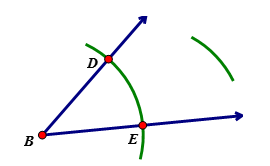3.5 In addition to Score 3.0 performance, the student demonstrates in-depth inferences and applications regarding the more complex content with partial success. Proficient “The Standard.” The student demonstrates no major errors or omissions regarding any of the information and processes that were end of instruction expectations. Construct a geometric figure using various tools and/or methods  (Ex: compass and straightedge, Geogebra) (a) copy a given line segment   (b) copy an angle. (c) bisect a line segment. (d) bisect an angle. (e) construct a line perpendicular to a given line through a point on that line. (f) construct a line perpendicular to a given line through a point not on that line. (g) construct the perpendicular bisector of a line segment. (h) construct a line parallel to a given line through a point not on the line.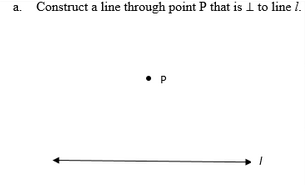Identify and complete the construction.2.5 The student demonstrates no major errors or omissions regarding the simpler details and processes (Score 2.0 content) and partial knowledge of the more complex ideas and processes (Score 3.0 content). Progressing The student demonstrates no major errors or omissions regarding the simpler details and processes but exhibits major errors or omissions regarding the more complex ideas and processes (Score 3.0 content). can recognize and recall terminology to answer questions  identify the type of construction given an example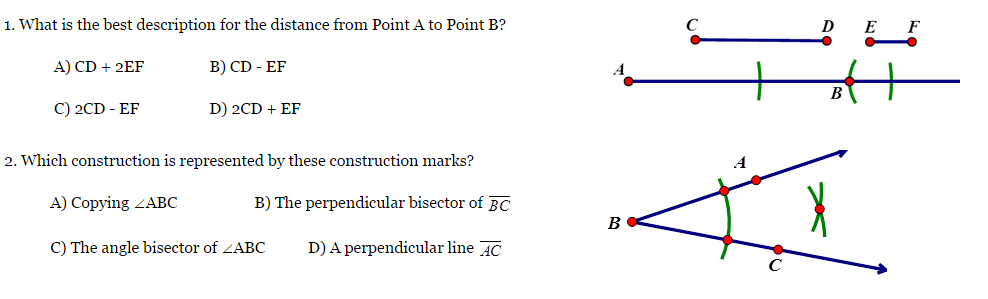1.5 The student demonstrates partial knowledge of the simpler details and processes (Score 2.0 content) but exhibits major errors or omissions regarding the more complex ideas and procedures (Score 3.0 content). Beginning With help, the student demonstrates a partial understanding of some of the simpler details and processes (Score 2.0 content) and some of the more complex ideas and processes (Score 3.0 content). - 0.5 With help, the student demonstrates a partial understanding of some of the simpler details and processes (Score 2.0 content) but not the more complex ideas and processes (Score 3.0 content). 0.0 Even with help, the student demonstrates no understanding or skill. -

## Resources

 Web Vocab

#### MAT-HS.G-CO.13

 MAT-HS Targeted Standards(G) Concept: Geometry(CO) Domain: CongruenceCluster: Make geometric constructions MAT-HS.G-CO.13 Construct an equilateral triangle, a square, and a regular hexagon inscribed in a circle.

## Student Learning Targets:

• I can
• I can

• I can
• I can

### Skills (Performance) Targets

• I can construct an equilateral triangle inscribed in a circle.
• I can construct a square inscribed in a circle.
• I can construct a regular hexagon inscribed in a circle.

• I can
• I can

## Proficiency Scale

 Score Description Sample Activity 4.0 In addition to Score 3.0, the student demonstrates in-depth inferences and applications regarding more complex material that go beyond end of instruction expectations. - 3.5 In addition to Score 3.0 performance, the student demonstrates in-depth inferences and applications regarding the more complex content with partial success. 3.0 “The Standard.” The student demonstrates no major errors or omissions regarding any of the information and processes that were end of instruction expectations. - 2.5 The student demonstrates no major errors or omissions regarding the simpler details and processes (Score 2.0 content) and partial knowledge of the more complex ideas and processes (Score 3.0 content). 2.0 The student demonstrates no major errors or omissions regarding the simpler details and processes but exhibits major errors or omissions regarding the more complex ideas and processes (Score 3.0 content). - 1.5 The student demonstrates partial knowledge of the simpler details and processes (Score 2.0 content) but exhibits major errors or omissions regarding the more complex ideas and procedures (Score 3.0 content). 1.0 With help, the student demonstrates a partial understanding of some of the simpler details and processes (Score 2.0 content) and some of the more complex ideas and processes (Score 3.0 content). - 0.5 With help, the student demonstrates a partial understanding of some of the simpler details and processes (Score 2.0 content) but not the more complex ideas and processes (Score 3.0 content). 0.0 Even with help, the student demonstrates no understanding or skill. -

## Resources

 Web Vocab

#### MAT-HS.G-GMD.01

 MAT-HS Targeted Standards(G) Concept: Geometry(GMD) Domain: Geometric Measurement and DimensionCluster: Explain volume formulas and use them to solve problems. MAT-HS.G-GMD.01 Give an informal argument for the formulas for the circumference of a circle, area of a circle, volume of a cylinder, pyramid, and cone. Use dissection arguments, Cavalieri’s principle, and informal limit arguments.

## Student Learning Targets:

• I can
• I can

### Reasoning Targets

• I can explain the formulas for circumference of a circle and area of a circle by determining the meaning of each term.
• I can explain the formulas for volume of a cylinder, pyramid and cone by determining the meaning of each term.

• I can
• I can

• I can
• I can

## Proficiency Scale

 Score Description Sample Activity 4.0 In addition to Score 3.0, the student demonstrates in-depth inferences and applications regarding more complex material that go beyond end of instruction expectations. - 3.5 In addition to Score 3.0 performance, the student demonstrates in-depth inferences and applications regarding the more complex content with partial success. 3.0 “The Standard.” The student demonstrates no major errors or omissions regarding any of the information and processes that were end of instruction expectations. - 2.5 The student demonstrates no major errors or omissions regarding the simpler details and processes (Score 2.0 content) and partial knowledge of the more complex ideas and processes (Score 3.0 content). 2.0 The student demonstrates no major errors or omissions regarding the simpler details and processes but exhibits major errors or omissions regarding the more complex ideas and processes (Score 3.0 content). - 1.5 The student demonstrates partial knowledge of the simpler details and processes (Score 2.0 content) but exhibits major errors or omissions regarding the more complex ideas and procedures (Score 3.0 content). 1.0 With help, the student demonstrates a partial understanding of some of the simpler details and processes (Score 2.0 content) and some of the more complex ideas and processes (Score 3.0 content). - 0.5 With help, the student demonstrates a partial understanding of some of the simpler details and processes (Score 2.0 content) but not the more complex ideas and processes (Score 3.0 content). 0.0 Even with help, the student demonstrates no understanding or skill. -

## Resources

 Web Vocab

#### MAT-HS.G-GMD.02

 MAT-HS Targeted Standards(G) Concept: Geometry(GMD) Domain: Geometric Measurement and DimensionCluster: Explain volume formulas and use them to solve problems.  MAT-HS.G-GMD.02 Calculate the surface area for prisms, cylinders, pyramids, cones, and spheres to solve problems.

• I can
• I can

• I can
• I can

• I can
• I can

• I can
• I can

## Proficiency Scale

 Score Description Sample Activity Advanced In addition to Score 3.0, the student demonstrates in-depth inferences and applications regarding more complex material that go beyond end of instruction expectations. Create a solid of revolution by rotating a 2 dimensional figure about a line and find the surface area of that solid. A geometric figure is created when bounded by the lines y=¾ x-3, y=0, x=0.  Draw a picture of the region being revolved about the x-axis.  Determine the surface area of the geometric solid. 3.5 In addition to Score 3.0 performance, the student demonstrates in-depth inferences and applications regarding the more complex content with partial success. Proficient “The Standard.” The student demonstrates no major errors or omissions regarding any of the information and processes that were end of instruction expectations. Calculate the surface area formulas for cylinders, pyramids, cones, and spheres to solve problems Solve for various heights and lengths for use in the surface area formulas using inductive reasoning, pythagorean theorem, and trigonometry. Label the answer using the correct units based on the context of the problem. Give either the approximate or exact answer, given the context of the problem. Find the surface area of the figure: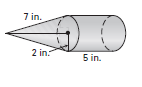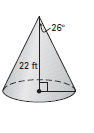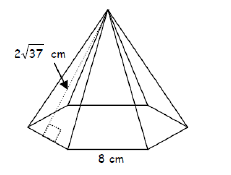2.5 The student demonstrates no major errors or omissions regarding the simpler details and processes (Score 2.0 content) and partial knowledge of the more complex ideas and processes (Score 3.0 content). Progressing The student demonstrates no major errors or omissions regarding the simpler details and processes but exhibits major errors or omissions regarding the more complex ideas and processes (Score 3.0 content). Select the appropriate formula for finding the surface area of a solid Students can solve for surface area of given shapes when given all necessary measurements Find the surface area of the figure: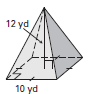1.5 The student demonstrates partial knowledge of the simpler details and processes (Score 2.0 content) but exhibits major errors or omissions regarding the more complex ideas and procedures (Score 3.0 content). Beginning With help, the student demonstrates a partial understanding of some of the simpler details and processes (Score 2.0 content) and some of the more complex ideas and processes (Score 3.0 content). can solve for surface area with help - 0.5 With help, the student demonstrates a partial understanding of some of the simpler details and processes (Score 2.0 content) but not the more complex ideas and processes (Score 3.0 content). 0.0 Even with help, the student demonstrates no understanding or skill. -

## Resources

 Web Vocab

#### MAT-HS.G-GMD.03

 MAT-HS Targeted Standards(G) Concept: Geometry(GMD) Domain: Geometric Measurement and DimensionCluster: Explain volume formulas and use them to solve problems MAT-HS.G-GMD.03 Know and apply volume formulas for prisms, cylinders, pyramids, cones, and spheres to solve problems.

## Student Learning Targets:

### Knowledge Targets

• I can choose the appropriate formula from a list.

### Reasoning Targets

• I can, given formulas, compute the surface area and volume of a right prism, right cylinder, right pyramid, right cone, and sphere.

• I can
• I can

• I can
• I can

## Proficiency Scale

 Score Description Sample Activity Advanced In addition to Score 3.0, the student demonstrates in-depth inferences and applications regarding more complex material that go beyond end of instruction expectations. Create a solid of revolution by rotating a 2 dimensional figure about a line and find the volume of that solid. A geometric figure is created when bounded by the lines y=¾ x-3, y=0, x=0.  Draw a picture of the region being revolved about the x-axis.  Determine the volume of the geometric solid. 3.5 In addition to Score 3.0 performance, the student demonstrates in-depth inferences and applications regarding the more complex content with partial success. Proficient “The Standard.” The student demonstrates no major errors or omissions regarding any of the information and processes that were end of instruction expectations. Know and use volume formulas for cylinders, pyramids, cones, and spheres to solve problems. Solve for various heights and lengths for use in the volume formulas using inductive reasoning, pythagorean theorem, and trigonometry. Label the answer using the correct units based on the context of the problem. Give either the approximate or exact answer, given the context of the problem. Find the volume of the figure:Find the value of x.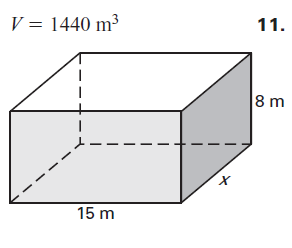2.5 The student demonstrates no major errors or omissions regarding the simpler details and processes (Score 2.0 content) and partial knowledge of the more complex ideas and processes (Score 3.0 content). Progressing The student demonstrates no major errors or omissions regarding the simpler details and processes but exhibits major errors or omissions regarding the more complex ideas and processes (Score 3.0 content). Select the appropriate formula for finding the volume of a solid. Students can solve for volume of given shapes when given all necessary measurements Find the volume of the figure:1.5 The student demonstrates partial knowledge of the simpler details and processes (Score 2.0 content) but exhibits major errors or omissions regarding the more complex ideas and procedures (Score 3.0 content). Beginning With help, the student demonstrates a partial understanding of some of the simpler details and processes (Score 2.0 content) and some of the more complex ideas and processes (Score 3.0 content). Students can solve for volume with help. 0.5 With help, the student demonstrates a partial understanding of some of the simpler details and processes (Score 2.0 content) but not the more complex ideas and processes (Score 3.0 content). 0.0 Even with help, the student demonstrates no understanding or skill. -

## Resources

 Web Vocab

#### MAT-HS.G-GMD.04

 MAT-HS Targeted Standards(G) Concept: Geometry(GMD) Domain: Geometric Measurement and DimensionCluster: Visualize relationships between two-dimensional and three-dimensional objects MAT-HS.G-GMD.04 Identify the shapes of two-dimensional cross-sections of three-dimensional objects, and identify three-dimensional objects generated by rotations of two-dimensional objects.

## Student Learning Targets:

### Knowledge Targets

• I can identify the properties and attributes of two and three-dimensional objects that distinguish one from another; e.g., a square is a parallelogram with four congruent sides and four right angles; a cylinder has two parallel circular bases.
• I can identify two dimensional cross sections of three dimensional objects.
• I can identify three dimensional objects created by rotating two dimensional objects.

• I can
• I can

• I can
• I can

• I can
• I can

## Proficiency Scale

 Score Description Sample Activity 4.0 In addition to Score 3.0, the student demonstrates in-depth inferences and applications regarding more complex material that go beyond end of instruction expectations. - 3.5 In addition to Score 3.0 performance, the student demonstrates in-depth inferences and applications regarding the more complex content with partial success. 3.0 “The Standard.” The student demonstrates no major errors or omissions regarding any of the information and processes that were end of instruction expectations. - 2.5 The student demonstrates no major errors or omissions regarding the simpler details and processes (Score 2.0 content) and partial knowledge of the more complex ideas and processes (Score 3.0 content). 2.0 The student demonstrates no major errors or omissions regarding the simpler details and processes but exhibits major errors or omissions regarding the more complex ideas and processes (Score 3.0 content). - 1.5 The student demonstrates partial knowledge of the simpler details and processes (Score 2.0 content) but exhibits major errors or omissions regarding the more complex ideas and procedures (Score 3.0 content). 1.0 With help, the student demonstrates a partial understanding of some of the simpler details and processes (Score 2.0 content) and some of the more complex ideas and processes (Score 3.0 content). - 0.5 With help, the student demonstrates a partial understanding of some of the simpler details and processes (Score 2.0 content) but not the more complex ideas and processes (Score 3.0 content). 0.0 Even with help, the student demonstrates no understanding or skill. -

## Resources

 Web Vocab

#### MAT-HS.G-GPE.01

 MAT-HS Targeted Standards(G) Concept: Geometry(GPE) Domain: Expressing Geometric Properties with EquationsCluster: Translate between the geometric description and the equation for a conic section MAT-HS.G-GPE.01 Derive the equation of a circle of given center and radius using the Pythagorean Theorem; complete the square to find the center and radius of a circle given by an equation.

## Student Learning Targets:

• I can
• I can

• I can
• I can

### Skills (Performance) Targets

• I can use the Pythagorean Theorem to derive the equation of a circle, given the center and radius. I can given an equation of a circle, complete the square to find the center and radius.

• I can
• I can

## Proficiency Scale

 Score Description Sample Activity 4.0 In addition to Score 3.0, the student demonstrates in-depth inferences and applications regarding more complex material that go beyond end of instruction expectations. - 3.5 In addition to Score 3.0 performance, the student demonstrates in-depth inferences and applications regarding the more complex content with partial success. 3.0 “The Standard.” The student demonstrates no major errors or omissions regarding any of the information and processes that were end of instruction expectations. - 2.5 The student demonstrates no major errors or omissions regarding the simpler details and processes (Score 2.0 content) and partial knowledge of the more complex ideas and processes (Score 3.0 content). 2.0 The student demonstrates no major errors or omissions regarding the simpler details and processes but exhibits major errors or omissions regarding the more complex ideas and processes (Score 3.0 content). - 1.5 The student demonstrates partial knowledge of the simpler details and processes (Score 2.0 content) but exhibits major errors or omissions regarding the more complex ideas and procedures (Score 3.0 content). 1.0 With help, the student demonstrates a partial understanding of some of the simpler details and processes (Score 2.0 content) and some of the more complex ideas and processes (Score 3.0 content). - 0.5 With help, the student demonstrates a partial understanding of some of the simpler details and processes (Score 2.0 content) but not the more complex ideas and processes (Score 3.0 content). 0.0 Even with help, the student demonstrates no understanding or skill. -

## Resources

 Web Vocab

#### MAT-HS.G-GPE.02

 MAT-HS Targeted Standards(G) Concept: Geometry(GPE) Domain: Expressing Geometric Properties with EquationsCluster: Translate between the geometric description and the equation for a conic section MAT-HS.G-GPE.02 Derive the equation of a parabola given a focus and directrix.

## Student Learning Targets:

### Knowledge Targets

• I can, given a parabola, identify the vertex, focus, directrix, and axis of symmetry, noting that every point on the parabola is equidistant from the focus and the directrix.

• I can
• I can

### Skills (Performance) Targets

• I can, given a focus and directrix, derive the equation of a parabola.

• I can
• I can

## Proficiency Scale

 Score Description Sample Activity 4.0 In addition to Score 3.0, the student demonstrates in-depth inferences and applications regarding more complex material that go beyond end of instruction expectations. - 3.5 In addition to Score 3.0 performance, the student demonstrates in-depth inferences and applications regarding the more complex content with partial success. 3.0 “The Standard.” The student demonstrates no major errors or omissions regarding any of the information and processes that were end of instruction expectations. - 2.5 The student demonstrates no major errors or omissions regarding the simpler details and processes (Score 2.0 content) and partial knowledge of the more complex ideas and processes (Score 3.0 content). 2.0 The student demonstrates no major errors or omissions regarding the simpler details and processes but exhibits major errors or omissions regarding the more complex ideas and processes (Score 3.0 content). - 1.5 The student demonstrates partial knowledge of the simpler details and processes (Score 2.0 content) but exhibits major errors or omissions regarding the more complex ideas and procedures (Score 3.0 content). 1.0 With help, the student demonstrates a partial understanding of some of the simpler details and processes (Score 2.0 content) and some of the more complex ideas and processes (Score 3.0 content). - 0.5 With help, the student demonstrates a partial understanding of some of the simpler details and processes (Score 2.0 content) but not the more complex ideas and processes (Score 3.0 content). 0.0 Even with help, the student demonstrates no understanding or skill. -

## Resources

 Web Vocab

Page: (Previous)   1  2  3  4  5  6  (Next)
ALL